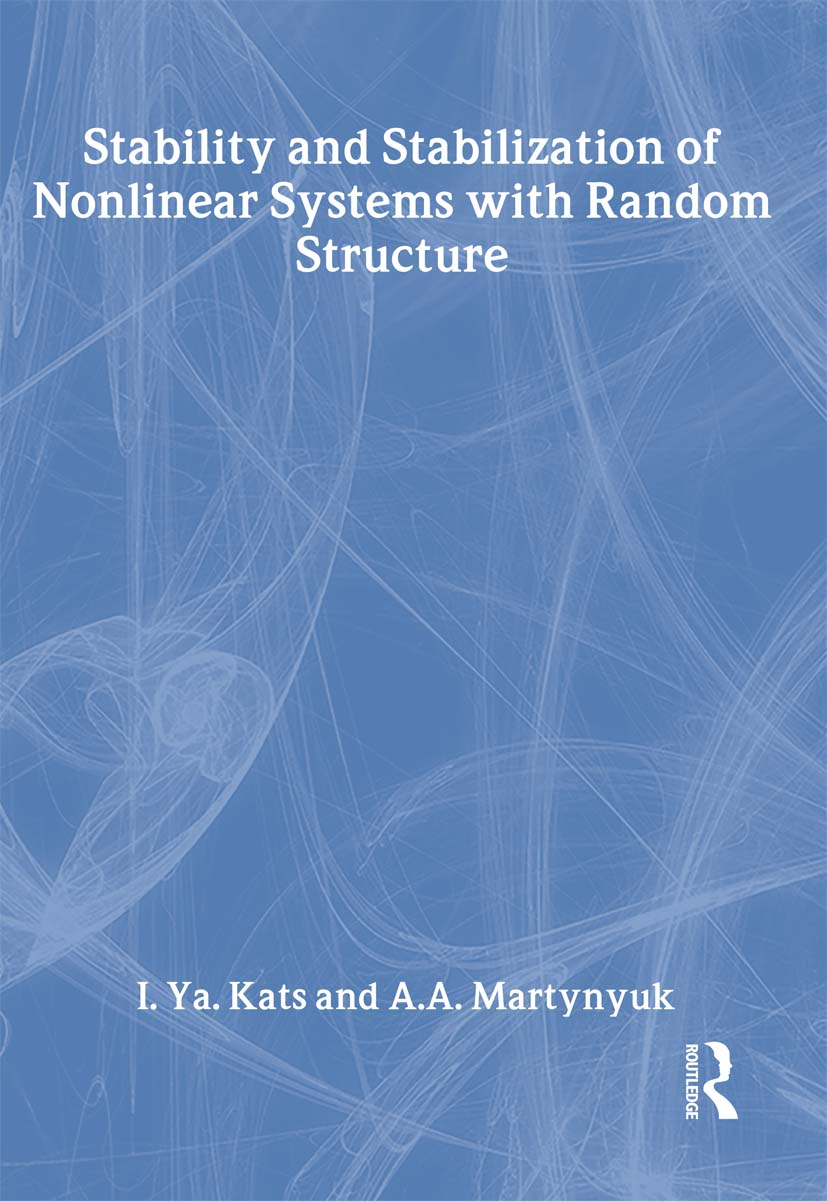# Stability and Stabilization of Nonlinear Systems with Random Structures

## 1st Edition

CRC Press

256 pages

##### Purchasing Options:\$ = USD
Hardback: 9780415272537
pub: 2002-08-22
SAVE ~\$47.00
Currently out of stock
\$235.00
\$188.00
x

FREE Standard Shipping!

### Description

Nonlinear systems with random structures arise quite frequently as mathematical models in diverse disciplines. This monograph presents a systematic treatment of stability theory and the theory of stabilization of nonlinear systems with random structure in terms of new developments in the direct Lyapunov's method. The analysis focuses on dynamic systems with random Markov parameters. This high-level research text is recommended for all those researching or studying in the fields of applied mathematics, applied engineering, and physics-particularly in the areas of stochastic differential equations, dynamical systems, stability, and control theory.

### Reviews

"This volume will be of interest to researchers and students in stochastic stability theory."

- Zentralblatt fur Mathematik, Vol. 1026

Introductory Remarks. Random Variables and Probability Distributions. Probability Processes and their Mathematical Description. Random Differential Equations. System with Random Structure. Stability Analysis Using Scalar Lyapunov Functions. Stability Concepts for Stochastic Systems. Random Scalar Lyapunov Functions. Conditions of Stability in Probability. Converse Theorems. Stability in Mean Square. Stability in Mean Square of Linear Systems. Stability Analysis Using Multi-component Lyapunov Functions. Vector Lyapunov Functions. Stochastic Matrix-Valued Lyapunov Functions. Stability Analysis in General. Stability Analysis of Systems in Ito's Form. Stochastic Singularly Perturbed Systems. Large-Scale Singularly Perturbed Systems. Stability Analysis by the First-Order Approximation. Stability Criterion by the First-Order Approximation. Stability with Respect to the First-Order Approximation. Stability by First-Order Approximation of Systems with Random Delay. Convergence of Stochastic Approximation Procedure. Stabilization of Controlled Systems with Random Structure. Problems of Stabilization. Optimal Stabilization. Linear-Quadratic Optimal Stabilization. Sufficient Stabilization Conditions for Linear Systems. Optimal Solution Existence. The Small Parameter Method Algorithm. Applications. A Stochastic Version of the Lefschetz Problem. Stability in Probability of Oscillating Systems. Stability in Probability of Regulation Systems. Price Stability in a Stochastic Market Model. References. Index.

Lyapunov Functions. Stability Analysis Using Multicomponent Lyapunov Functions. Stability Analysis by the First-order Approximation. Stabilization of Controlled Systems with Random Structure. Applications; References; Index.

### Subject Categories

##### BISAC Subject Codes/Headings:
MAT003000
MATHEMATICS / Applied
MAT007000
MATHEMATICS / Differential Equations